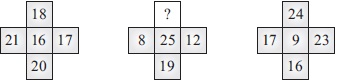# User Forum

Subject :IMO    Class : Class 8

The characters in given figures follows a certain rule row-wise or column-wise. Identify the rule and find the missing number.A1
B8
C16
D9

## Ans 1:

Class : Class 10
Also 18+20 = 21+17=38 in Figure 1 In figure 2 1+19 = 12+8 = 20 In figure 3 24+16 = 17+23 = 40

## Ans 2:

Class : Class 10
I think the middle part was given just to confuse us

Class : Class 10